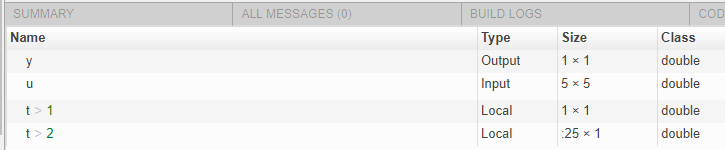Reuse the Same Variable with Different Properties

When You Can Reuse the Same Variable with Different Properties

You can reuse (reassign) an input, output, or local variable with different class, size, or complexity if the code generator can unambiguously determine the properties of each occurrence of this variable during C/C++ code generation. If so, MATLAB® creates separate uniquely named local variables in the generated code. You can view these renamed variables in the code generation report.

A common example of variable reuse is in if-elseif-else or switch-case statements. For example, the following function example1 first uses the variable t in an if statement, where it holds a scalar double, then reuses t outside the if statement to hold a vector of doubles.

function y = example1(u) %#codegen
if all(all(u>0))
% First, t is used to hold a scalar double value
t = mean(mean(u)) / numel(u);
u = u - t;
end
% t is reused to hold a vector of doubles
t = find(u > 0);
y = sum(u(t(2:end-1)));

When You Cannot Reuse Variables

You cannot reuse (reassign) variables if it is not possible to determine the class, size, and complexity of an occurrence of a variable unambiguously during code generation. In this case, variables cannot be renamed and a compilation error occurs.

For example, the following example2 function assigns a fixed-point value to x in the if statement and reuses x to store a matrix of doubles in the else clause. It then uses x after the if-else statement. This function generates a compilation error because after the if-else statement, variable x can have different properties depending on which if-else clause executes.

function y = example2(use_fixpoint, data) %#codegen
if use_fixpoint
% x is fixed-point
x = fi(data, 1, 12, 3);
else
% x is a matrix of doubles
x = data;
end
% When x is reused here, it is not possible to determine its
% class, size, and complexity
t = sum(sum(x));
y = t > 0;
end

Example 3. Variable Reuse in an if Statement

To see how MATLAB renames a reused variable t:

1. Create a MATLAB file example1.m containing the following code.

function y = example1(u) %#codegen
if all(all(u>0))
% First, t is used to hold a scalar double value
t = mean(mean(u)) / numel(u);
u = u - t;
end
% t is reused to hold a vector of doubles
t = find(u > 0);
y = sum(u(t(2:end-1)));
end

2. Generate a MEX function for example1 and produce a code generation report.

codegen -o example1x -report example1.m -args {ones(5,5)}

3. Open the code generation report.

On the Variables tab, you see two uniquely named local variables t>1 and t>2.4. In the list of variables, click t>1. The report highlights the instances of the variable t that are inside of the if statement. These instances of t are scalar double.

5. Click t>2. The code generation report highlights the instances of t that are outside of the if statement. These instances of t are variable-size column vectors with an upper bound of 25.

Limitations of Variable Reuse

The following variables cannot be renamed in generated code:

• Persistent variables.

• Global variables.

• Variables passed to C code using coder.ref, coder.rref, coder.wref.

• Variables whose size is set using coder.varsize.

• The index variable of a for-loop when it is used inside the loop body.

• The block outputs of a MATLAB Function block in a Simulink® model.

• Chart-owned variables of a MATLAB function in a Stateflow® chart.

Fixed-Point Designer DocumentationGet trial now